# Uncertainty in Physical Measurements Module 6

Uncertainty Module 6 - UM Student Guide Aug. 8, 2016, 1:11 p.m.

## Miscellaneous Topics

This Module discusses some miscellaneous topics that don’t fit nicely into the previous Modules. The Sections in this Module are:

1. Confidence Intervals

2. Uncertainty in a Count

3. Systematic Effects and Calibration

4. Outliers and Robust Estimators

5. Accepted Values

## 1 - Confidence Intervals

We have seen that the standard uncertainty assigned to a measurement says that there is some probability that the true value of some quantity is within plus or minus the uncertainty of the measured value. For a rectangular probability distribution the probability is 0.58, for a triangular distribution it is 0.65, and for a Gaussian it is 0.68. These probabilities define a confidence interval. We say that the confidence levels are 58%, 65%, and 68% for these three different pdfs.

For a Gaussian probability distribution function with centre value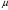, the area under the curve between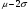and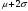is 0.95, so reporting the uncertainty to be twice the standard deviation defines a 95% confidence interval. Similarly, three standard deviations defines a 99% confidence interval.

In many fields of science, the 95% confidence interval is the standard. For example, in 1992 Mackowiak, Wasserman, and Levine measured the body temperature of 130 healthy adults.  Note that this is a U.S. study so the temperatures are all in Fahrenheit. Most people believe that a healthy person’s temperature is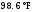. The question they were trying to answer was whether this is correct. The mean value of their measured temperatures was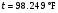with a standard deviation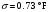, which was larger than the uncertainties in the reading and accuracy of the thermometer. The standard uncertainty in the mean was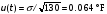. Therefore the 95% confidence interval was: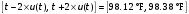(1)

Since a Gaussian only approaches zero asymptotically at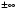, there is no finite width that has an area equal to one, so for a true Gaussian pdf there is always a non-zero chance that the true value is not within the claimed uncertainty of the measured value. For a 95% confidence interval, that chance is 5%. Eqn 1 indicates that there is only a 5% chance that the standard temperature ofis the correct value.

As you may know, a complex and difficult experiment based at CERN in Switzerland has recently reported the detection of the Higgs boson, the so-called “God particle.” That research group required that the data were consistent with detection of the Higgs to five standard deviations before they would publish their results. The chance that the Higgs was not actually observed works out to be 1 part in 3,500,000. This very strict criterion is often called a “5 sigma” requirement.

In principle, we can also define 95% confidence intervals for rectangular and triangular probability distribution functions by including a wider range of values under the curve than the area defined by the standard deviation, although in practice this is seldom actually done.

## 2 - Uncertainty in a Count

Way back in Module 1 we were rolling a pair of dice 36 times. We will now think about rolling a seven. The probability of rolling a seven is 1/6, so the theoretical prediction is that we should get 6 sevens after 36 rolls. Except there is also a non-zero probability that when you rolled the dice 36 times you only got 5 sevens, or maybe 9 sevens, or maybe even 0 sevens.

You may recognise this as an example of a binomial distribution. For completeness, if we have N trials with a probability p for a particular result, the probability of that result occurring n times is given by: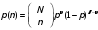(2)

where: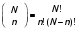(3)

and the exclamation mark ! means factorial.

The dots in Figure 1 shows the probability distribution function for our dice: it is the binomial distribution, Eqns. 2 and 3, with N = 36, p = 1/6 and n is the number of times we got a seven.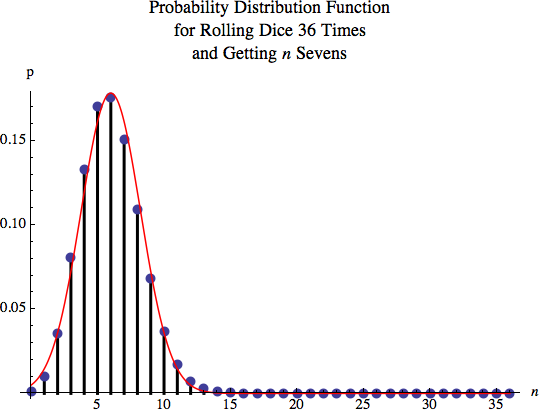#### Figure 1

The highest probability is for n = 6, the theoretical prediction, and p(n = 6) = 0.18. The probability of getting 5 sevens is almost is high, p(n = 5) = 0.17. The probability of getting 0 sevens is pretty small, p(n = 0) = 0.0014, and the probability of getting 36 sevens is miniscule,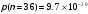. For n < 0 and n > 36, the probabilities are exactly 0.

Since the binomial pdf is not symmetric, the mean is not the mid-point of the distribution. The mean of the binomial pdf can be shown to be Np, which for our example is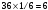. The standard deviation can be shown to be: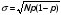(4)

The solid curve in Figure 1 is a Gaussian pdf with the same mean and standard deviation: it is clearly a reasonable approximation of the binomial pdf. This justifies something that we explored in Module 4, when we realized that for many datasets assuming a Gaussian distribution is only an approximation. Here we see that it can be a very good approximation.

Imagine that some Team, maybe yours, rolled the dice 36 times and got 4 sevens. The probability of this occurring is p(n = 4) = 0.13. The question is: what is the uncertainty in the value of 4? If a large number of Teams roll the dice 36 times, about 13% of them should have gotten 4 sevens. From Eqn. 4 the uncertainty is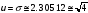. Therefore we can write the result as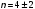, and the result is within the given uncertainty of the theoretical prediction of 6 sevens.

This is a general property of an integer value that is the result of counting:

If we count n things and there are statistical fluctuations in the data, a good first guess of the statistical uncertainty in the value of n is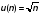.

##Questions

1. In the strike-shortened 2012-2013 NHL season, Phil Kessel of the Toronto Maple Leafs scored 20 goals. Some were “lucky” goals and at other times he had excellent scoring chances that failed to find the net. What is the statistical uncertainty in the number of goals he scored?

2. For the Physics test marks shown in Module 4 Figure 5, there were 65 marks between 60% and 69% (C), and 46 marks between 70% and 79% (B). Were there significantly more C’s than B’s on the test?

3. In Module 1 you were asked to devise a reasonable measure of whether or not your results for rolling dice were close to the theoretical prediction. Can you now replace your answer with a better one? If so, what is it? We are not asking you to go back and re-do you that Activity.

## Example

Suppose that someone decides to investigate whether jellybeans cause cancer. She assembles a large body of people, half of whom she feeds jellybeans to, and half she does not. The sample that ate no jellybeans is the control group. She continues the study for some years. At the end she compares cancer rates for the two groups, and finds that they are the same within uncertainties at the 95% confidence level. She reports that jellybeans do not cause cancer.

She decides to extend her study by using different flavoured jellybeans. There are 16 common flavours of jellybeans, so she assembles 17 different samples of people. The control group ate no jellybeans, and each of the other groups ate a single flavour of jellybeans. After some years she compares cancer rates for the 17 groups. She finds no difference within uncertainties for 16 of the groups: the control group and all the other groups except the ones who ate the pink wintergreen flavor. The cancer rate for the group who ate wintergreen jellybeans was higher than the control group who ate no jellybeans at a 95% confidence level. The next day all the newspapers had front page stories with this headline:

WINTERGREEN FLAVOUR JELLYBEANS CAUSE CANCER!

Only 5% Chance of Coincidence!

However, there is a big problem with this result. We are comparing 16 different jellybean-eating groups to the control group. Assume that none of the flavours actually cause cancer. Then what is the probability that all of the 16 jellybean-eating samples had measured cancer rates that were same as the control group within 95% confidence level uncertainties? This is another application of the binomial distribution and the probability works out to be only 0.56. So there is a significant chance that one (or more) flavours gave a result that was different than the control group, either giving a rate of cancer formation that was higher or lower than the control.

## 3 – Systematic Effects and Calibration

Every experimentalist fears that there are systematic effects that make their results wrong. We saw an example of such systematic errors in Module 0, when we discussed the OPERA team’s report that the speed of neutrinos was greater than the speed of light by 6 standard deviations. Later they discovered that there was a loose fiber optic cable and an incorrectly functioning oscillator that meant that the result was wrong.

Sometimes systematic effects lead us to new discoveries. For example, in the late 19th century Lord Rayleigh measured the mass of one liter of nitrogen of two different samples, both at the same pressure and temperature. One sample used nitrogen isolated from the atmosphere, and the other from nitrogen prepared from a chemical reaction. Although Rayleigh didn’t calculate the uncertainties, his results were: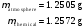(5)

These two values differ from each other by only about 0.5%. Rayleigh thought the difference was significant, and that there was some impurity in the chemical sample. Sir William Ramsay believed it was an impurity in the atmospheric sample. Ramsay turned out to be correct, and together they identified the impurity: it was the then unknown element Argon. This was the first noble gas that was isolated. For this work Rayleigh received a Nobel Prize in physics and Ramsay received a Nobel Prize in chemistry.

Often systematic effects are due to the instruments that we use to make a measurement. For example, we might have a voltmeter that always reads voltages that are 20% too high. Often we can calibrate the instrument to minimise or even eliminate these effects. We saw an example of a calibration in Module 2, when we measured a temperature with an instrument that reads to the tenth of a degree, such as 12.8, and measure the same temperature with a better instrument that measures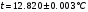. Then we know the temperature is within 0.003 of a degree of 12.820 when the first thermometer reads 12.8.

Another example could be an experiment in measuring positions with a webcam connected to a computer. We take a photograph of a checkerboard with a webcam such as Figure 2. Although the checkerboard is square, the 3-dimensional perspective means that the image of the checkerboard is not perfectly square. Then we can calibrate the x and y pixels in the photograph to the known x, y, and z positions of the checkerboard.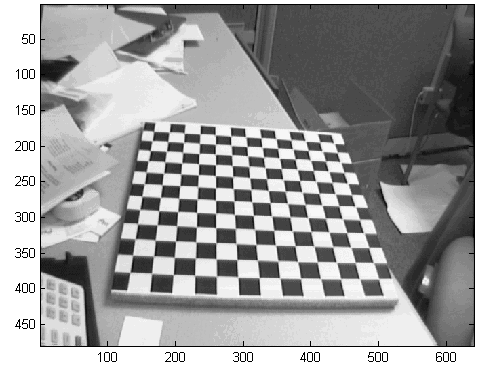##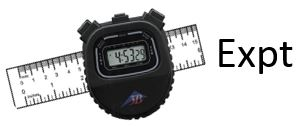Activity 1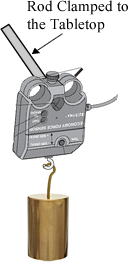In this Activity you will calibrate a Pasco Economy Force Sensor Model CI-6746. The manufacturer does not specify an accuracy for the instrument. The sensor is mounted to the tabletop, and you will suspend masses from the hook on the sensor. The sensor is wired to the U of T Data Acquisition box, which connects to the computer. On the computer you will want to use the ForceSensor program in the LabVIEW Shortcuts folder on your desktop. To start the software, click on the Acquire button in the upper-left. You can read the force read by the sensor, in N, in the digital display in the lower-right.

The force exerted on the sensor when the mass m is hanging from it is mg. You may assume that g = 9.801 m/s2. You will want to use the balance in the room to measure the value of the mass that you hang from the sensor.

Notice that the bottoms of the masses have a hole with another hook, so you can combine two or more masses to hang from the sensor.

Your goal in the calibration is to be able to report what the actual force is for any given reading on the computer. This will necessarily involve accounting for the uncertainty in the force that is applied because of the uncertainties in measuring the masses with the balance. For some values of the force as read by the software, the display may jitter between two values, and that will lead to an uncertainty in the values you read for the sensor, which also needs to be accounted for.

Also, the Force Sensor tends to drift, so press the TARE button on the sensor before each measurement.

If you wish to try to fit your data to some model, such as a line, you can create a dataset with the CreateDataset program in the LabVIEW Shortcuts folder, and then fit it with the PolynomialFit program in the same folder. If you wish to fit to a straight line but force the line to go through the origin, fit to a power 1 but not a power 0: this forces the intercept to be zero.

## 4 – Outliers and Robust Estimators

Sometimes when we collect data, one datapoint seems far away from the expected value as determined by the other values. Here we will think about such data and how to deal with it. In particular, can we throw away a datapoint because it is an outlier? This is an awkward question.

Imagine we have repeated some measurement ten times and get the values shown in Table 1.

 Trial Value 1 1.4 2 1.3 3 1.4 4 101.3 5 1.5 6 1.5 7 1.6 8 1.5 9 1.6 10 1.4

#### Table 1

The 4th measurement appears to be an outlier. Should we just throw it out? Perhaps that value indicates some unknown physical process, and often we learn about new science by figuring out what process led to an apparent outlier. So dropping a suspect datapoint is always dangerous. There are various ways of trying to objectively answer the question of whether or not to drop an outlier, of which the best known is Chauvenet’s Criterion. However, all such methods are subject to intense and justified criticism by many.

The mean of all the data of Table 1 is 11.45, but if we drop the 4th datapoint the mean of the other 9 values is 1.47. This indicates a problem with all calculations of the mean: it is not robust and a single wild datapoint can seize control of the result. The median is the value for which ½ of the values are less than the median and ½ of the values are greater than the median. The median is robust: so long as less than one-half of the values are “contaminated” it will not give an arbitrarily large result. The median for all 10 datapoints in Table 1 is the same as the value for the 9 datapoints after dropping the 4th one: 1.5.

The standard deviation, which measures the width of a distribution, is also not robust. For the data of Table 1, the standard deviations of all the datapoints and the data with the 4th one dropped are 31.6 and 0.1 respectively. A robust way of measuring the width of a distribution is the quartiles. The first quartile is the value for which ¼ of the data is less than the value and ¾ of the data is greater. The second quartile is the median. The third quartile is the value for which ¼ of the data is greater than the value and ¾ of the data is less. The first quartiles for the data of Table 1, with and without the 4th datapoint, are both 1.4. The third quartiles are almost the same: 1.6 and 1.525 respectively. The interquartile range (IQR) is the value of the third quartile minus the value of the first quartile, and is a measure of the full width of the distribution. The half-width of the distribution is one-half of the interquartile range. Therefore, just as we previously wrote that the value and uncertainty of the mean of N measurements can be given by: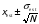(6)

we can use robust estimators to give the median and its uncertainty as: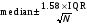(7)

Assigning a probability to the uncertainty in Eqn. 7 is difficult, since the interquartile range suppresses data that may be outliers.

##Activity 2

Imagine that you wish to determine the acceleration due to gravity g using a mass on a string. The mass is oscillating with a small maximum amplitude. The model, from Newton’s Laws, is that the period T of the mass is related to the length from the pivot point to the centre of the mass L and g by: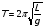(8)

so: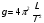(9)

Your experimental procedure is to measure the time for 5 oscillations, t5, so the period is t5/5. You calculate that the total uncertainty in each measurement due to the reading uncertainty and the accuracy uncertainty of the stopwatch is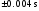. You repeat the measurement ten times, and the results are shown in Table 2.

 Trial t5 (s) 1 7.52 2 7.52 3 7.37 4 6.12 5 7.45 6 7.37 7 7.56 8 7.51 9 7.50 10 7.50

#### Table 2

The 4th datapoint appears to be an outlier. For your convenience, we have prepared a Python program that defines the values of Table 2 in a variable named t5. The program also defines a “trimmed” dataset of the value of Table 2 with the 5th data point omitted: the trimmed dataset is named t5t. For your convenience, we have also calculated the means, standard deviations, lengths, medians, and quartiles for both datasets. The program also prints the standard deviations and the interquartile ranges.

Print the estimated mean and calculate and print its uncertainty from Eqn. 6 for the t5 data. You will want to know that the Python function to calculate square roots is sqrt(). Print the median and its uncertainty from Eqn. 7 for the t5 data.

Next print the results for the mean and the median, including the uncertainties for the t5t data.

Can you think of any reason why the spread of values as measured by the interquartile range of both sets of data or of the standard deviation of the trimmed data is so much larger than the uncertainty of? If so, what is it?

Often when dealing with an outlier, this sort of analysis is about as far as we can go. Perhaps when you were taking the 4th datapoint a gravity wave came through the room. But for determining g you don’t want to use the gravity-wave influenced measurement, although discovering a gravity wave could be a huge amount of fun. However, for this data you may be able to think of some reason why the 4th datapoint is out of line with the others. Can you think of any such reason? If so, what is it? Hint: review Question 3 from Module 3.

Define a new list, naming it perhaps t5c for t4 corrected, with a corrected 4th datapoint. Calculate the estimated mean and median and their uncertainties.

So, what is your best estimate of the value and uncertainty in the value of the period T? Do you need to worry about the uncertainty in the values of t5 because of the reading uncertainty and accuracy uncertainty, which above we stated was? Explain.

Fitting data with an outlier to a model using least-squares techniques is similar to calculating the mean: an outlier can seize control of the fit. This turns out to be for the same reason: the least-squares method involves calculating means. You saw an example of an outlier seizing a fit in Activity 2 of Module 5, where fitting the third of the Anscombe datasets to a straight line gave the fit shown in Figure 3.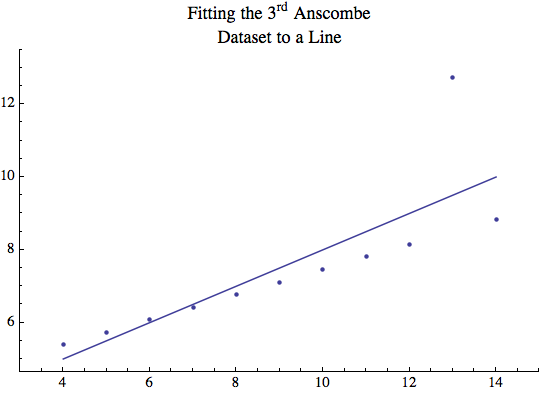#### Figure 3

Although 10 of the 11 datapoints lie along a nice straight line, the one outlier has made the fit silly. As you showed, the slope of the line is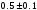and the intercept is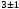.

A possible way to deal with data like this is to use robust estimators. We define two partitions that divide the data into 3 datasets each with roughly the same number of datapoints. For each partition we calculate the medians of both the independent and dependent variables. Then we can do a least-squares straight line fit to the medians. Figure 4 shows the result of this procedure. The slope is 0.35 and the intercept is 4.0. It is difficult to know how to calculate uncertainties in these values, which is one problem with this technique. Nonetheless, we clearly see that the outlier is being ignored by the fit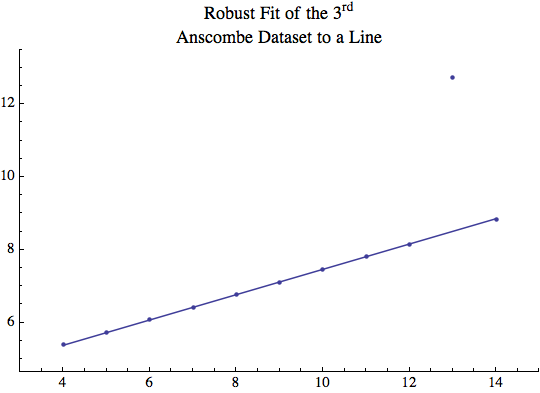#### Figure 4

A final example involves 14C dating, which depends on the ratio of 14C to C in the atmosphere. However, this ratio has changed over time and the exact value for any given time is not well known. For coral in the sea, a more precise and accurate method to find the coral’s age involves thorium dating with a mass spectrometer. Figure 5 shows a calibration of 14C dating: the independent variable is the age of some corals off Barbados as determined by 14C dating and the dependent variable is the result of thorium dating minus the 14C value. ( E. Bard, B. Hamelin, R.G. Fairbanks, and A. Zindler, “Calibration of the 14C timescale over the past 30,000 years using mass spectrometer U-TH ages from Barbados corals,” Nature 345 (1990), 405.) If the 14C data were correct, the value of the dependent variable should be zero within uncertainties.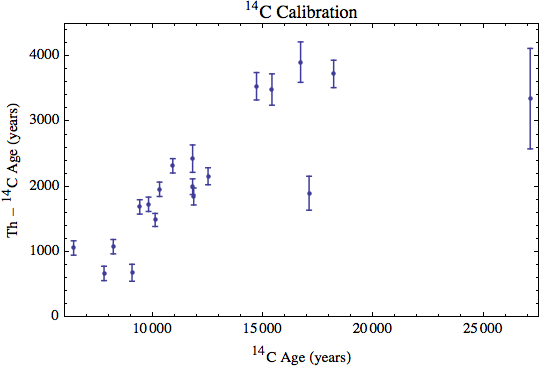#### Figure 5

In general the discrepancy increases more-or-less linearly with the 14C value, but there are two outliners at 14C ages of 17,085 and 27,120 years. The question is should these two values be discarded? Perhaps they are just wrong datapoints. Or perhaps they reflect some local effect that only applies off the coast of Barbados, where the data were taken. Or perhaps they reflect some global phenomenon around those years and should be used in all 14C calibrations. These questions cannot really be answered without lots more investigation of the data than is available.

However, we can sort of “squint our eyes” and just do a robust straight line fit to the data, although this ignores the important questions about why the “outliers” are there. The result is shown in Figure 6.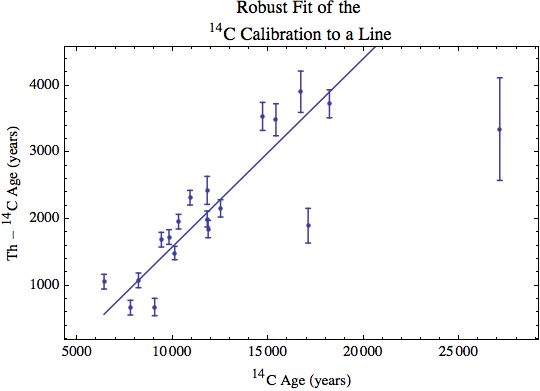## 5 – Accepted Values

Sometimes there is some value of a physical quantity that most people believe is correct. This is called the accepted value. The value can change as new experiments are performed. For example, Newton’s Law of Gravitation is given by: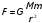(10)

where G is a universal constant. Here are the results of some experiments to determine its value:

 Experiment G (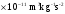) Cavendish (1798)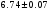Luther and Taylor (1982)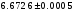Parks and Fuller (2010)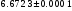Quinn, Parks, Speake, and Davis (2013)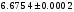#### Table 3

You may wish to notice that the 2013 result is not within uncertainties of the 1982 or 2010 ones, so there is some controversy about the “true” value of G.

In Activity 2 in the previous Section, we were considering using an oscillating mass to determine the acceleration due to gravity g. The model was given by Eqn. 9, which we write again:If the uncertainty in the period is u(T) and the uncertainty in the length is u(L), then propagating the uncertainties as we learned about in Module 4 gives an uncertainty in the value of g, u(g), that is: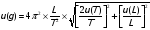(10)

In the Activity, we didn’t quite complete the experiment because we didn’t supply the value and uncertainty of the length of the pendulum L. Nonetheless, this is the only experiment that we have considered in these Modules for which there is an accepted value of the result, the value of g. It is: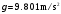(11)

There are some problems with this value. Among the difficulties are that g depends on:

• the distance from the centre of the Earth.

• the latitude. This is because the Earth is rotating on its axis and also because the Earth is not a perfect sphere.

• whether there are any nearby concentrations of high-density of low-density masses.

For example,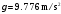in Mexico City and 9.825 m/s2 in Oslo. The WolframAlpha web site quotes a value for Toronto of 9.80678 m/s2, although you may not believe the number of significant figures in this number.

##Questions

4. Here are the results of four different determinations of g from the period of four different pendulums in Toronto: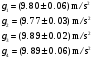(12)

Comment on these results. Are any of them wrong? Are any of them correct? Which is the best result? How can you tell?

5. Verify that Eqn. 10 is correct.Some teaching laboratories actually assess students on how closely their experimental result matches the accepted value. This is almost always not appropriate. A correct result of an experiment is the result when the experiment and data analysis have been performed correctly.

## Summary of Names, Symbols, and Formulae

Confidence Interval: a range of values which indicates the reliability of an estimate for a given probability expressed in percent.

Confidence Level: the probability that the value of the measurand is within the confidence interval.

Binomial Distribution: a description of the probability of measuring a result of repeating a measurement with the result having a given probability.

Uncertainty in a Count: if a count N objects and there are statistical uncertainties, the best estimate of the uncertainty in the count is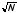.

Calibration: checking and possibly correcting the result of a measurement.

Outlier: one of more datapoints whose value does not appear to be consistent with the other datapoints in the dataset.

Robust: a value which is not greatly influenced by the presence of outliers in the data.

Using robust estimators:

Median: a value that separates the higher half of a dataset from the lower half.

Quartiles: a ranked set of 3 values that divide a dataset into four equally sized groups. The first quartile divides the lower 25% of the data from the higher 75%. The second quartile is the median. The third quartile divides the lower 75% of the data from the higher 25%.

Interquartile Range (IQR): the 3rd quartile minus the 1st quartile.

A robust estimate of the value and uncertainty of a dataset of N repeated measurements:Partition: a value of the independent variable that divides a dataset into two parts, or sometimes the divided dataset itself.

Accepted value: the value of some physical quantity that most people accept as being correct.

## Python Code for Activity 2

from pylab import *
from scipy import *

# This is the t5 data

t5 = [7.52, 7.52, 7.37, 6.12, 7.45, 7.37, 7.56, 7.51, 7.50, 7.50]

# This is the "trimmed" data: the 4th value is omitted. Note the "t"
# at the end of the name of the 2nd variable which stands for "trimmed"

t5t = [7.52, 7.52, 7.37, 7.45, 7.37, 7.56, 7.51, 7.50, 7.50]

# Calculate the mean of t5 and the mean of t5t.
# The name "avg" is for "average" and the name
# "avgt" is for the average of the trimmed data

avg = mean(t5)
avgt = mean(t5t)

# Calculate the standard deviations. Again, the trailing "t"
# in the second variable is for the trimmed datas

sd = std(t5, ddof = 1)
sdt = std(t5t, ddof = 1)

# The lengths of the lists

n = len(t5)
nt = len(t5t)

# Calculate the first quartiles.  The "25" means 25%

q1 = percentile(t5, 25)
q1t = percentile(t5t, 25)

# Calculate the medians.  We could also use percent( list , 50)

med = median(t5)
medt = median(t5t)

# Calculate the third quartiles, i.e. percent = 75%

q3 = percentile(t5, 75)
q3t = percentile(t5t, 75)


This Guide was written by David M. Harrison, Dept. of Physics, Univ. of Toronto, September 2013.

Modified by David M. Harrison, October 25, 2013; November 4, 2014.